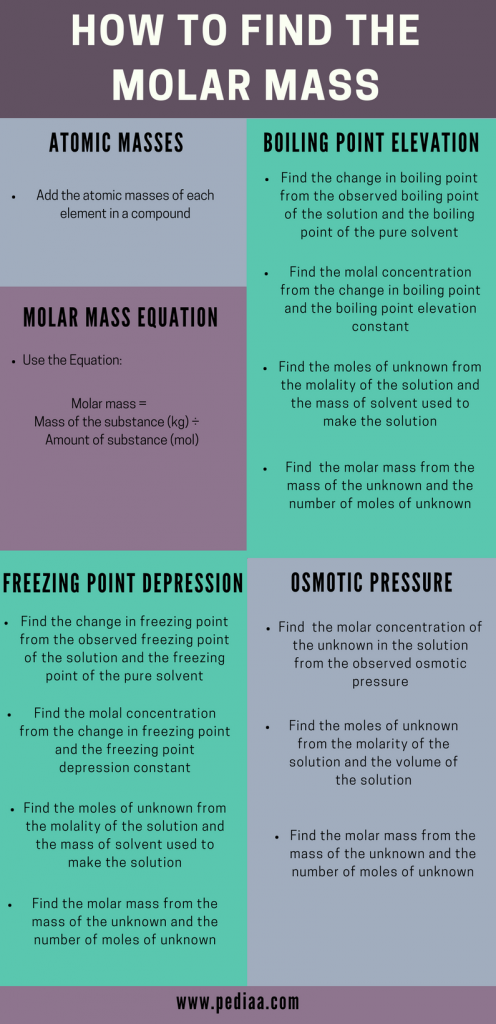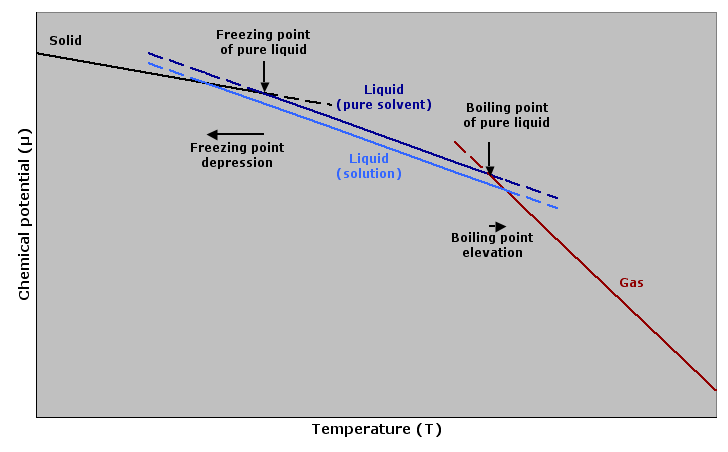# How to Find Molar Mass

Molar mass is a physical property of substances. It is very useful in analyzing, comparing and predicting the other physical and chemical properties such as density, melting point, boiling point, and the amount of substance that react with another substance in a system. There is more than one method to calculate the molar mass. Some of these methods include using the direct equation, adding the atomic masses of different elements in a compound, and using boiling point elevation or freezing point depression. Some of these major methods will be discussed concisely in this article.

### Key Areas Covered

1. What is Molar Mass
– Definition, Equation for Calculation, Explanation
2. How to Find Molar Mass
– Methods to Determine the Molar Mass
3.What is the Importance of Knowing the Molar Mass of a Substance
– Applications of Molar Mass

Key Terms: Avogadro’s Number, Boiling Point, Calusius-Clapeyron, Cryoscopic Constant, Ebullioscopic Constant, Freezing Point, Melting Point, Molality, Molar Mass, Molecular Weight, Osmotic Pressure, Relative Atomic Mass## What is Molar Mass

Molar mass is the mass of a mole of a particular substance. The most commonly used unit for the molar mass of a substance is gmol-1. However, the SI unit for molar mass is kgmol-1 (or kg/mol). The molar mass can be calculated using the following equation.

Molar Mass = Mass of the Substance (Kg)/Amount of Substance (Mol)

Mole or mol is the unit used to measure the amount of a substance. One mole of a substance is equal to a very large number, 6.023 x 1023 of atoms (or molecules) which the substance is made of. This number is called the Avogadro’s number. It is a constant because no matter what the type of atom is, one mole of it is equal to that amount of atoms (or molecules). Therefore, the molar mass can be given a new definition, that is, molar mass is the total mass of 6.023 x 1023 atoms (or molecules) of a particular substance. To avoid confusion, take a look at the following example.

• Compound A is composed of A molecules.
• Compound B is composed of B molecules.
• One mole of compound A is composed of 6.023 x 1023 of A molecules.
• One mole of compound B is composed of 6.023 x 1023 of B molecules.
• Molar mass of compound A is the sum of masses of 6.023 x 1023 A molecules.
• Molar mass of compound B is the sum of masses of 6.023 x 1023 B molecules.

Now we can apply this for real substances. One mole of H2O is composed of 6.023 x 1023 H2O molecules. The total mass of 6.023 x 1023 H2O molecules is about 18 g. Therefore, the molar mass of H2O is 18 g/mol.

## How to Find Molar Mass

The molar mass of a substance can be calculated using several methods such as;

1. Using atomic masses
2. Using the equation for calculating the molar mass
3. From boiling point elevation
4. From freezing point depression
5. From osmotic pressure

These methods are discussed in detail below.

### Using Atomic Masses

The molar mass of a molecule can be determined using atomic masses. This can be done simply by the addition of molar masses of each atom present. Molar mass of an element is given as below.

Molar mass of an element    = Relative atomic mass x molar mass constant (g/mol)

Relative atomic mass is the mass of an atom relative to the mass of Carbon-12 atom and it has no units. This relationship can be given as follows.

Molecular weight of A = Mass of one molecule of A/ [Mass of one carbon-12 atom x (1/12)]

Let’s consider following examples to understand this technique. Followings are the calculations for compounds with the same atom, combination of several different atoms and combination of a large number of atoms.

• Molar Mass of H2

o Types of atoms present     = Two H atoms
o Relative atomic masses     = 1.00794 (H)
o Molar mass of each atom  = 1.00794 g/mol (H)
o Molar mass of compound = (2 x 1.00794) g/mol
= 2.01588 g/mol

• Molar Mass of HCl

o Types of atoms present     = One H atom and one Cl atom
o Relative atomic masses     = 1.00794 (H) + 35.453 (Cl)
o Molar mass of each atom  = 1.00794 g/mol (H) + 35.453 g/mol (Cl)
o Molar mass of compound = (1 x 1.00794) + (1 x 35.453) g/mol
= 36.46094 g/mol

• Molar mass of C6H12O6

o Types of atoms present     = 6 C atoms, 12 H atoms and 6 O Cl atom
o Relative atomic masses     = 12.0107 (C) + 1.00794 (H) + 15.999 (O)
o Molar mass of each atom  = 12.0107 g/mol + 1.00794 g/mol (H) + 15.999 g/mol (O)
o Molar mass of compound = (6 x 12.0107) + (12 x 1.00794) + (6 x 15.999) g/mol
= 180.15348 g/mol

### Using the Equation

The molar mass can be calculated using the equation given below. This equation is used to determine an unknown compound. Consider the following example.

Molar mass =  Mass of the substance (kg)/Amount of substance (mol)

• The compound D is in a solution. The details are given as follows.
• Compound D is a strong base.
• It can release one H+ ion per molecule.
• The solution of compound D was made using 0.599 g of compound D.
• It reacts with HCl in the ratio of 1: 1

Then the determination can be done by an acid-base titration. Since it is a strong base, titrate the solution with a strong acid (Ex: HCl, 1.0 mol/L) in the presence of phenolphthalein indicator. The color change indicates the end point (Ex: when 15.00mL of HCl is added) of the titration and now all the molecules of the unknown base are titrated with the added acid. Then the molar mass of the unknown compound can be determined as follows.

o The amount of acid reacted                     = 1.0 mol/L x 15.00 x 10-3 L
= 1.5 x 10-2 mol
o Therefore, the amount of base reacted = 1.5 x 10-2 mol
o The molar mass of compound D            = 0.599 g / 1.5 x 10-2 mol
= 39.933 g/mol
o Then the unknown compound D can be predicted as NaOH. (But in order to confirm this, we should do further analysis).

### From Boiling Point Elevation

Boiling point elevation is the phenomenon which describes that the addition of a compound to a pure solvent would increase the boiling point of that mixture to a higher boiling point than that of the pure solvent. Therefore, the molar mass of that added compound can be found using the temperature difference between two boiling points. If the boiling point of the pure solvent is Tsolvent and the boiling point of the solution (with the added compound) is Tsolution, the difference between two boiling points can be given as below.

ΔT = Tsolution – Tsolvent

With the use of Clausius-Clapeyron relation and Raoult’s law, we can get a relationship between ΔT and molality of the solution.

ΔT = Kb . M

Where Kb is ebullioscopic constant and depend only on the properties of the solvent and M is the molality

From the above equation, we can get a value for molality of the solution. As the amount of solvent used for the preparation of this solution is known, we can find the value for moles of the compound added.

Molality = Moles of compound added (mol)/Mass of pure solvent used (kg)

Now that we know the moles of compound in the solution and the mass of the compound added, we can determine the molar mass of the compound.

Molar mass = Mass of compound (g)/Moles of compound (mol)Figure 01: Boiling Point Elevation and Freezing Point Depression

### From Freezing Point Depression

Freezing point depression is the opposite of boiling point elevation. Sometimes, when a compound is added to a solvent, the freezing point of the solution is lowered than that of the pure solvent. Then the above equations are a little bit modified.

ΔT = Tsolution – Tsolvent

The ΔT value is a minus value because the boiling point is now lower than the initial value. The molality of the solution can be obtained the same as in boiling point elevation method.

ΔT = Kf . M

Here, the Kf  is known as the cryoscopic constant. It is dependent only on the properties of the solvent.

The rest of the calculations are the same as in boiling point elevation method. Here, the moles of the compound added can also be calculated using the below equation.

Molality = Moles of compound (mol)/ Mass of solvent used (kg)

Then the molar mass can be calculated using the value for moles of compound added and the mass of compound added.

Molar mass = Mass of compound (g)/Moles of compound (mol)

### From Osmotic Pressure

Osmotic pressure is the pressure needed to be applied to avoid a pure solvent from passing to a given solution by osmosis. The osmotic pressure can be given in below equation.

∏  =  MRT

Where, ∏ is the osmotic pressure,
M is the molarity of the solution
R is the universal gas constant
T is the temperature

The molarity of the solution is given by the following equation.

Molarity = Moles of compound (mol) / Volume of solution (L)

The volume of the solution can be measured and the molarity can be calculated as above. Therefore, the moles of the compound in the solution can be measured. Then the molar mass can be determined.

Molar mass = Mass of compound (g)/Moles of compound (mol)

## What is the Importance of Knowing the Molar Mass of a Substance

• Molar masses of different compounds can be used to compare the melting points and boiling points of those compounds.
• Molar mass is used to determine the mass percentages of atoms present in a compound.
• Molar mass is very important in chemical reactions to find out the amounts of a certain reactant that has reacted or to find the amount of the product that can be obtained.
• Knowing the molar masses is very important before an experimental set up is designed.

### Summary

There are several methods to calculate the molar mass of a given compound. The easiest way among them is the addition of molar masses of elements present in that compound.

##### References:

1. “Mole.” Encyclopædia Britannica. Encyclopædia Britannica, inc., 24 Apr. 2017. Web. Available here. 22 June 2017.
2. Helmenstine, Anne Marie. “How to Calculate Molar Mass.” ThoughtCo. N.p., n.d. Web. Available here. 22 June 2017.
3. Robinson, Bill. “Determining molar mass.” Chem.purdue.edu. N.p., n.d. Web. Available here. 22 June 2017.
4. “Freezing Point Depression.” Chemistry LibreTexts. Libretexts, 21 July 2016. Web. Available here 22 June 2017.

##### Image Courtesy:

1. “Freezing point depression and boiling point elevation” By Tomas er – Own work (CC BY-SA 3.0) via Commons Wikimedia